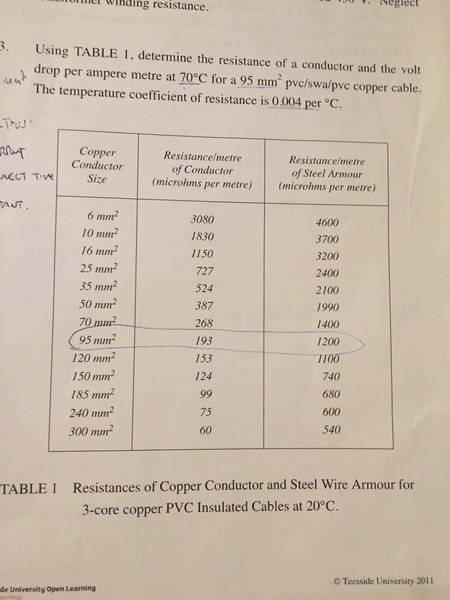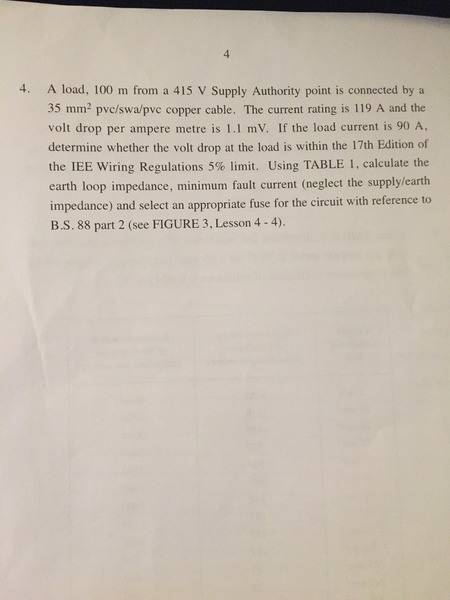# How to find volt drop per ampere meter?

• Neil Hayes
In summary: different metals with the same coefficient of resistance at the same temperature would seem contradictory to me.

## Homework Statement

[/B]
Determine the resistance of a conductor and the volt
drop per ampere metre at 70°C for a 95 mm2 pvc/swa/pvc copper cable.
The temperature coefficient of resistance is 0.004 per °C.

## Homework Equations

[/B]
R70 ( resistance at 70 degrees ) = R20 [ 1+( temperature coefficient of resistance of copper X's temperature difference ) ]

## The Attempt at a Solution

[/B]
Hi there folks!

I have the above question as part of work I'm doing on a course that I'm studying.

I've found what the resistance/meter is by using the following equation:

R70 ( resistance at 70 degrees ) = R20 [ 1+( temperature coefficient of resistance of copper X temperature difference ) ]

I was provided with a table which showed the resistance/meter for cables at 20 degrees.

My notes have in all examples given the volt drop per ampere per meter so I'm not 100% sure on how to obtain it myself. I'm thinking that this resistance is the same value as volt drop per ampere per meter as using ohms law V = I x's R and therefore 1 amp multiplied by R70 from above equation should give a volt drop per ampere per meter.

Is my thinking correct?

Any thoughts would be much appreciated!

Neil

By Ohm's Law, R = V/I means that 1 Ohm = 1 Volt per Amphere.

Hi Simon,

In my lessons the volt drop per ampere per meter value is always given. In this question I have been given no current or voltage but I have worked out the resistance of the 95 mm² cable per meter at 70 degrees. Seen as voltage is current times resistance I was thinking that the volt drop per ampere per meter would be ( I x's R ) so I would multiply my found resistance per meter by 1 to give me a volt drop per ampere per meter.

Please review post #2. There is nothing complicated or mysterious here. 1 Ohm = 1 Volt per Amp.
This means that the "Ohm" and the "Volt per Amp" are different ways fo writing the same thing.
So everywhere you see Ω just replace it with VA-1

You could say that to turn Ohms into Volts per Amp you use the conversion factor 1VA-1Ω-1
S if you have 10Ω and you want to know what that is in VA-1 you do: (10Ω)*(1VA-1Ω-1)=10VA-1
I mean - if you really wanted to. Mathematically the scale factor between the two is 1 so why bother?

This means that if you have Ohms per meter, then that is already Volts per Amp per meter.
Just by replacing every instance of "Ohms" with "Volts per Amp".

By the same token, if you have Ohms per carrot, then that is already Volts per Amp per carrot.

•Neil Hayes
Hi again Simon,

Thanks for the reply ( again )! All makes sense. Thanks for your help clearing up my confusionNeil

Hi there, i have the same question and also found this a little confusing as Neil Hayes.

So i have calculated my R70 as
R20x[(1+0.004x50)]
R20 = From table 1 193 + 1200 ( 193 is the resistance/meter of conductor 1200 is the resistance/meter of steel armour. Both given in Table 1)
so 1393 x 1.2 = 1671.6 microhms meter
therefore volt drop = 0.0016716A per meter
Any help would be greatly welcomed
Thanks Tom

Tom McCormack said:
Hi there, i have the same question and also found this a little confusing as Neil Hayes.

So i have calculated my R70 as
R20x[(1+0.004x50)]
R20 = From table 1 193 + 1200 ( 193 is the resistance/meter of conductor 1200 is the resistance/meter of steel armour. Both given in Table 1)
so 1393 x 1.2 = 1671.6 microhms meter
therefore volt drop = 0.0016716A per meter
Any help would be greatly welcomed
Thanks Tom
Wouldn't the steel jacket be in parallel with the copper conductor?
Why would the same temperature coefficient apply to both metals?
Are you sure it isn't just the resistance of the copper conductor that's of interest?

gneill said:
Wouldn't the steel jacket be in parallel with the copper conductor?
Why would the same temperature coefficient apply to both metals?
Are you sure it isn't just the resistance of the copper conductor that's of interest?

I am not too sure i was working from the example in the learning material.
Thanks Tom

Tom McCormack said:
I am not too sure i was working from the example in the learning material.
Thanks Tom
I don't have the benefit of seeing your learning materials, so I can't comment on any example you're following. My thoughts are pretty much embodied in the questions I posed. When I think of a steel-armored copper cable I think of a central copper conductor surrounded by an insulating sheath, surrounded by a steel sleeve or braid, surrounded by more insulating material. I'd think that the steel wouldn't be in contact with the copper anywhere except possibly at the ends of the cable where a manual connection of some form would be required. Two different metals being in contact is usually a big no-no for corrosion and electrolytic activity (the junction acting like a crude battery and ensuing chemical reactions hastening corrosion).

My instincts would be to deal with the copper conductor alone.

If you can share the original text of the problem statement and perhaps a link to, or an image of, the relevant data table that might help.

gneill said:
I don't have the benefit of seeing your learning materials, so I can't comment on any example you're following. My thoughts are pretty much embodied in the questions I posed. When I think of a steel-armored copper cable I think of a central copper conductor surrounded by an insulating sheath, surrounded by a steel sleeve or braid, surrounded by more insulating material. I'd think that the steel wouldn't be in contact with the copper anywhere except possibly at the ends of the cable where a manual connection of some form would be required. Two different metals being in contact is usually a big no-no for corrosion and electrolytic activity (the junction acting like a crude battery and ensuing chemical reactions hastening corrosion).

My instincts would be to deal with the copper conductor alone.

If you can share the original text of the problem statement and perhaps a link to, or an image of, the relevant data table that might help.

Thanks again here is the questions i have.Thanks. I strongly suspect that they want you to consider the resistance of the copper conductor only for the first question.
I don't know enough about IEE Wiring Regulations to speculate on an approach to question #4, whether the armour shield becomes part of the ground loop wiring or not, or how the grounding is accomplished at either or both ends of the circuit.

gneill said:
Thanks. I strongly suspect that they want you to consider the resistance of the copper conductor only for the first question.
I don't know enough about IEE Wiring Regulations to speculate on an approach to question #4, whether the armour shield becomes part of the ground loop wiring or not, or how the grounding is accomplished at either or both ends of the circuit.

Thanks for the help
So in that case would you agree with me on

R70 as R20x[(1+0.004x50)] R20 = From table 1 193
so 193 x 1.2 = 231.6 microhms meter therefore volt drop = 0.0002316A per meter
Thanks again

Looks okay except for the voltage drop units. I suppose I'd quote the voltage drop per ampere-meter as ##232~\frac{μV}{A m}##, rounding to three significant figures.

gneill said:
Looks okay except for the voltage drop units. I suppose I'd quote the voltage drop per ampere-meter as ##232~\frac{μV}{A m}##, rounding to three significant figures.

Thanks for your helpNeil Hayes said:
I've found what the resistance/meter is by using the following equation:
Resistance per metre mixes a physical quantity with a unit of measure.
You may have either ohms/metre or resistance/length.